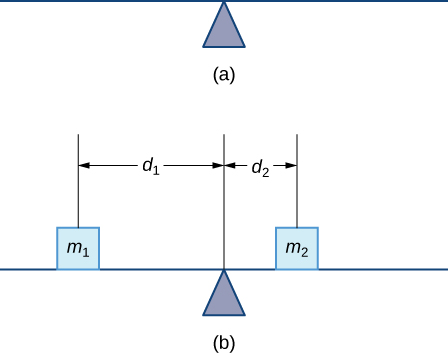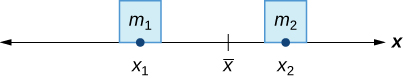# 6.6 Moments and centers of mass

 Page 1 / 14
• Find the center of mass of objects distributed along a line.
• Locate the center of mass of a thin plate.
• Use symmetry to help locate the centroid of a thin plate.
• Apply the theorem of Pappus for volume.

In this section, we consider centers of mass (also called centroids , under certain conditions) and moments. The basic idea of the center of mass is the notion of a balancing point. Many of us have seen performers who spin plates on the ends of sticks. The performers try to keep several of them spinning without allowing any of them to drop. If we look at a single plate (without spinning it), there is a sweet spot on the plate where it balances perfectly on the stick. If we put the stick anywhere other than that sweet spot, the plate does not balance and it falls to the ground. (That is why performers spin the plates; the spin helps keep the plates from falling even if the stick is not exactly in the right place.) Mathematically, that sweet spot is called the center of mass of the plate .

In this section, we first examine these concepts in a one-dimensional context, then expand our development to consider centers of mass of two-dimensional regions and symmetry. Last, we use centroids to find the volume of certain solids by applying the theorem of Pappus.

## Center of mass and moments

Let’s begin by looking at the center of mass in a one-dimensional context. Consider a long, thin wire or rod of negligible mass resting on a fulcrum, as shown in [link] (a). Now suppose we place objects having masses ${m}_{1}$ and ${m}_{2}$ at distances ${d}_{1}$ and ${d}_{2}$ from the fulcrum, respectively, as shown in [link] (b).(a) A thin rod rests on a fulcrum. (b) Masses are placed on the rod.

The most common real-life example of a system like this is a playground seesaw, or teeter-totter, with children of different weights sitting at different distances from the center. On a seesaw, if one child sits at each end, the heavier child sinks down and the lighter child is lifted into the air. If the heavier child slides in toward the center, though, the seesaw balances. Applying this concept to the masses on the rod, we note that the masses balance each other if and only if ${m}_{1}{d}_{1}={m}_{2}{d}_{2}.$

In the seesaw example, we balanced the system by moving the masses (children) with respect to the fulcrum. However, we are really interested in systems in which the masses are not allowed to move, and instead we balance the system by moving the fulcrum. Suppose we have two point masses, ${m}_{1}$ and ${m}_{2},$ located on a number line at points ${x}_{1}$ and ${x}_{2},$ respectively ( [link] ). The center of mass, $\stackrel{–}{x},$ is the point where the fulcrum should be placed to make the system balance.The center of mass x – is the balance point of the system.

Thus, we have

$\begin{array}{ccc}\hfill {m}_{1}|{x}_{1}-\stackrel{–}{x}|& =\hfill & {m}_{2}|{x}_{2}-\stackrel{–}{x}|\hfill \\ \hfill {m}_{1}\left(\stackrel{–}{x}-{x}_{1}\right)& =\hfill & {m}_{2}\left({x}_{2}-\stackrel{–}{x}\right)\hfill \\ \hfill {m}_{1}\stackrel{–}{x}-{m}_{1}{x}_{1}& =\hfill & {m}_{2}{x}_{2}-{m}_{2}\stackrel{–}{x}\hfill \\ \hfill \stackrel{–}{x}\left({m}_{1}+{m}_{2}\right)& =\hfill & {m}_{1}{x}_{1}+{m}_{2}{x}_{2}\hfill \\ \hfill \stackrel{–}{x}& =\hfill & \frac{{m}_{1}{x}_{1}+{m}_{2}{x}_{2}}{{m}_{1}+{m}_{2}}.\hfill \end{array}$

The expression in the numerator, ${m}_{1}{x}_{1}+{m}_{2}{x}_{2},$ is called the first moment of the system with respect to the origin. If the context is clear, we often drop the word first and just refer to this expression as the moment    of the system. The expression in the denominator, ${m}_{1}+{m}_{2},$ is the total mass of the system. Thus, the center of mass    of the system is the point at which the total mass of the system could be concentrated without changing the moment.

how does this work
Can calculus give the answers as same as other methods give in basic classes while solving the numericals?
log tan (x/4+x/2)
Rohan
Rohan
y=(x^2 + 3x).(eipix)
Claudia
Ismael
A Function F(X)=Sinx+cosx is odd or even?
neither
David
Neither
Lovuyiso
f(x)=1/1+x^2 |=[-3,1]
apa itu?
fauzi
determine the area of the region enclosed by x²+y=1,2x-y+4=0
Hi
MP
Hi too
Vic
hello please anyone with calculus PDF should share
Which kind of pdf do you want bro?
Aftab
hi
Abdul
can I get calculus in pdf
Abdul
How to use it to slove fraction
Hello please can someone tell me the meaning of this group all about, yes I know is calculus group but yet nothing is showing up
Shodipo
You have downloaded the aplication Calculus Volume 1, tackling about lessons for (mostly) college freshmen, Calculus 1: Differential, and this group I think aims to let concerns and questions from students who want to clarify something about the subject. Well, this is what I guess so.
Jean
Im not in college but this will still help
nothing
how can we scatch a parabola graph
Ok
Endalkachew
how can I solve differentiation?
with the help of different formulas and Rules. we use formulas according to given condition or according to questions
CALCULUS
For example any questions...
CALCULUS
v=(x,y) وu=(x,y ) ∂u/∂x* ∂x/∂u +∂v/∂x*∂x/∂v=1
log tan (x/4+x/2)
Rohan
what is the procedures in solving number 1?
review of funtion role?
for the function f(x)={x^2-7x+104 x<=7 7x+55 x>7' does limx7 f(x) exist?
find dy÷dx (y^2+2 sec)^2=4(x+1)^2
Integral of e^x/(1+e^2x)tan^-1 (e^x)
why might we use the shell method instead of slicing
fg[[(45)]]²+45⅓x²=100By Brooke DelaneyBy OpenStaxBy Mike WolfBy OpenStaxBy Anonymous UserBy Madison ChristianBy OpenStaxBy Zarina ChocolateBy JavaChamp TeamBy Richley Crapo